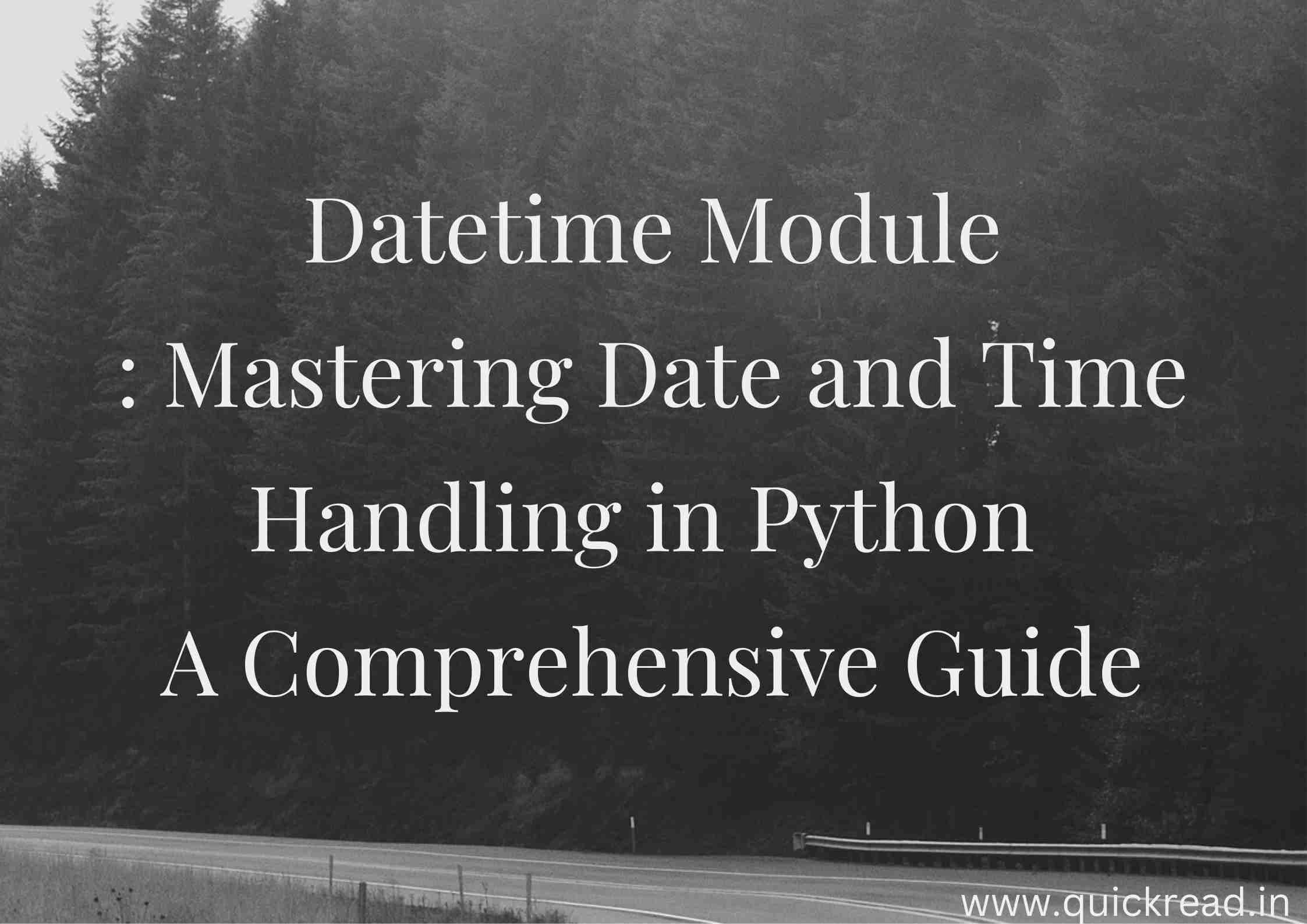## Introduction

Dates and times are a critical component of most applications. Python provides extensive support for handling dates and times in programs. In this tutorial, we will explore Python’s datetime module including objects, methods and common operations related to dates and times.

## Datetime Module

Python’s `datetime` module contains classes for manipulating dates and times. Some key classes are:

• date – Represents calendar date (year, month, day)
• time – Represents time of day independent of date
• datetime – Combines both date and time
• timedelta – Represents duration, time difference
• tzinfo – Abstract class for time zone info

These provide building blocks for date and time manipulations. Let’s look at them in more detail.

## Date Object in Python

A `date` object represents a calendar date with year, month and day attributes:

``````from datetime import date

d = date(2023, 2, 15)
print(d) # 2023-02-15

print('Year:', d.year) # 2023
print('Month:', d.month) # 2
print('Day:', d.day) # 15``````

`date.today()` can be used to get the current local date.

Dates support operations like comparisons to check which date is earlier:

``````d1 = date(2023, 3, 30)
d2 = date(2023, 3, 28)

print(d1 > d2) # True
print(d1 == d2) # False``````

Date arithmetic is also possible:

``````d3 = d1 - timedelta(days=2)
print(d3) # 2023-03-28``````

## Time Object in Python

A `time` object represents a time independent of day:

``````from datetime import time

t = time(11, 34, 58)
print(t) # 11:34:58
print(t.hour, t.minute, t.second) # 11 34 58``````

`time.now()` provides the current local time.

Times can be compared similar to dates:

``````t1 = time(9, 30)
t2 = time(10, 15)

print(t1 < t2) # True``````

Time arithmetic is also supported:

``````t3 = t1 + timedelta(hours=2, minutes=15)
print(t3) # 11:45:00``````

## Datetime Object in Python

`datetime` combines date and time objects into one:

``````from datetime import datetime

dt = datetime(2023, 3, 15, 10, 15, 30)
print(dt) # 2023-03-15 10:15:30

print(dt.date()) # Date component
print(dt.time()) # Time component``````

Now is obtained via `datetime.now()` and timezones can be attached:

``````dt_now = datetime.now()
dt_mtn = dt_now.astimezone(timezone('US/Mountain'))

print(dt_mtn) # 2023-03-01 07:35:24.639946-07:00``````

Datemath and comparisons work similarly on datetimes.

## Converting to Strings in Python

Dates and times can be converted to nicely formatted strings:

``````from datetime import datetime

dt = datetime(2023, 3, 1, 10, 0)

print(dt.strftime('%m/%d/%Y, %H:%M')) # 03/01/2023, 10:00
print(dt.isoformat()) # 2023-03-01T10:00:00``````

Custom string formats can be used like `%d/%m/%Y`. ISO format provides an unambiguous representation.

## Timedeltas in Python

`timedelta` represents a duration:

``````from datetime import timedelta

delta = timedelta(days=7, hours=12)
print(delta) # 7 days, 12:00:00

dt1 = datetime(2023, 3, 8, 10, 0)
dt2 = dt1 - delta

print(dt2) # 2023-03-01 10:00:00``````

Timedeltas are useful for date math and calculating differences.

## Handling timezone in Python

Here are some ways to handle timezones in Python:

• The datetime module provides some timezone support. The tzinfo class can be subclassed to provide timezone information.
• The pytz module offers higher level timezone handling capabilities. It contains timezone definitions and provides methods like localize(), normalize(), astimezone() etc. to convert datetimes between timezones.
• To make a datetime timezone aware in Python:
``````from datetime import datetime
from pytz import timezone

dt = datetime(2023, 1, 1, 12, 0, 0)
eastern = timezone('US/Eastern')
dt = eastern.localize(dt)``````

Once a datetime is timezone aware, it can be converted to other timezones:

``dt = dt.astimezone(timezone('Europe/London'))``

pytz is the de facto library for timezone handling in Python

## Conclusion

Python offers excellent support for date and time programming via its datetime module. date, time, datetime and timedelta objects provide flexible capabilities to handle common use cases like parsing, formatting, arithmetic and timezone conversions. Robust handling of dates and times is essential for most applications. Mastering datetime functionalities unlocks building powerful calendar driven programs in Python.

1. How do you get the current date and time in Python?

Use `datetime.now()` to get the current date and time. `datetime.today()` also available.

1. How are timezones handled in Python?

Python’s pytz module provides timezone handling. datetime can attach timezones.

1. What is the difference between datetime and timestamp?

Datetime represents absolute date and time. Timestamp is number of seconds since epoch.

1. How do you convert a string to datetime in Python?

Use `datetime.strptime()` to parse a formatted string into a datetime object.

1. How are timedeltas useful with datetimes in Python?

Timedeltas represent relative time differences. They are useful for date math like adding/subtracting intervals.